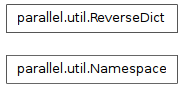parallel.factory

testing

# parallel.util¶

## Module: parallel.util¶

Inheritance diagram for IPython.parallel.util:some generic utilities for dealing with classes, urls, and serialization

Authors:

• Min RK

## Classes¶

### Namespace¶

class IPython.parallel.util.Namespace

Bases: dict

Subclass of dict for attribute access to keys.

__init__()

x.__init__(...) initializes x; see x.__class__.__doc__ for signature

clear

D.clear() -> None. Remove all items from D.

copy

D.copy() -> a shallow copy of D

static fromkeys(S[, v]) → New dict with keys from S and values equal to v.

v defaults to None.

get

D.get(k[,d]) -> D[k] if k in D, else d. d defaults to None.

has_key

D.has_key(k) -> True if D has a key k, else False

items

D.items() -> list of D’s (key, value) pairs, as 2-tuples

iteritems

D.iteritems() -> an iterator over the (key, value) items of D

iterkeys

D.iterkeys() -> an iterator over the keys of D

itervalues

D.itervalues() -> an iterator over the values of D

keys

D.keys() -> list of D’s keys

pop

D.pop(k[,d]) -> v, remove specified key and return the corresponding value. If key is not found, d is returned if given, otherwise KeyError is raised

popitem

D.popitem() -> (k, v), remove and return some (key, value) pair as a 2-tuple; but raise KeyError if D is empty.

setdefault

D.setdefault(k[,d]) -> D.get(k,d), also set D[k]=d if k not in D

update

D.update(E, **F) -> None. Update D from dict/iterable E and F. If E has a .keys() method, does: for k in E: D[k] = E[k] If E lacks .keys() method, does: for (k, v) in E: D[k] = v In either case, this is followed by: for k in F: D[k] = F[k]

values

D.values() -> list of D’s values

### ReverseDict¶

class IPython.parallel.util.ReverseDict(*args, **kwargs)

Bases: dict

simple double-keyed subset of dict methods.

__init__(*args, **kwargs)
clear

D.clear() -> None. Remove all items from D.

copy

D.copy() -> a shallow copy of D

static fromkeys(S[, v]) → New dict with keys from S and values equal to v.

v defaults to None.

get(key, default=None)
has_key

D.has_key(k) -> True if D has a key k, else False

items

D.items() -> list of D’s (key, value) pairs, as 2-tuples

iteritems

D.iteritems() -> an iterator over the (key, value) items of D

iterkeys

D.iterkeys() -> an iterator over the keys of D

itervalues

D.itervalues() -> an iterator over the values of D

keys

D.keys() -> list of D’s keys

pop(key)
popitem

D.popitem() -> (k, v), remove and return some (key, value) pair as a 2-tuple; but raise KeyError if D is empty.

setdefault

D.setdefault(k[,d]) -> D.get(k,d), also set D[k]=d if k not in D

update

D.update(E, **F) -> None. Update D from dict/iterable E and F. If E has a .keys() method, does: for k in E: D[k] = E[k] If E lacks .keys() method, does: for (k, v) in E: D[k] = v In either case, this is followed by: for k in F: D[k] = F[k]

values

D.values() -> list of D’s values

## Functions¶

IPython.parallel.util.asbytes(s)

ensure that an object is ascii bytes

IPython.parallel.util.connect_engine_logger(context, iface, engine, loglevel=10)
IPython.parallel.util.connect_logger(logname, context, iface, root='ip', loglevel=10)
IPython.parallel.util.disambiguate_ip_address(ip, location=None)

turn multi-ip interfaces ‘0.0.0.0’ and ‘*’ into connectable ones, based on the location (default interpretation of location is localhost).

IPython.parallel.util.disambiguate_url(url, location=None)

turn multi-ip interfaces ‘0.0.0.0’ and ‘*’ into connectable ones, based on the location (default interpretation is localhost).

This is for zeromq urls, such as tcp://*:10101.

IPython.parallel.util.generate_exec_key(keyfile)
IPython.parallel.util.integer_loglevel(loglevel)
IPython.parallel.util.interactive(f)

decorator for making functions appear as interactively defined. This results in the function being linked to the user_ns as globals() instead of the module globals().

IPython.parallel.util.local_logger(logname, loglevel=10)
IPython.parallel.util.pack_apply_message(f, args, kwargs, threshold=6.3999999999999997e-05)

pack up a function, args, and kwargs to be sent over the wire as a series of buffers. Any object whose data is larger than threshold will not have their data copied (currently only numpy arrays support zero-copy)

IPython.parallel.util.select_random_ports(n)

Selects and return n random ports that are available.

IPython.parallel.util.serialize_object(obj, threshold=6.3999999999999997e-05)

Serialize an object into a list of sendable buffers.

Parameters : obj : object The object to be serialized threshold : float The threshold for not double-pickling the content. (‘pmd’, [bufs]) : : where pmd is the pickled metadata wrapper, bufs is a list of data buffers
IPython.parallel.util.signal_children(children)

Relay interupt/term signals to children, for more solid process cleanup.

IPython.parallel.util.split_url(url)

split a zmq url (tcp://ip:port) into (‘tcp’,’ip’,’port’).

IPython.parallel.util.unpack_apply_message(bufs, g=None, copy=True)

unpack f,args,kwargs from buffers packed by pack_apply_message() Returns: original f,args,kwargs

IPython.parallel.util.unserialize_object(bufs)

reconstruct an object serialized by serialize_object from data buffers.

IPython.parallel.util.validate_url(url)

validate a url for zeromq

IPython.parallel.util.validate_url_container(container)

validate a potentially nested collection of urls.